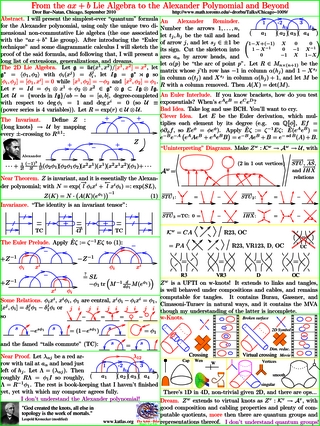© | << < ? > >> | Dror Bar-Natan: Talks:

# Two Talks in Chicago, September 2010

## Talk I: From the ax+b Lie Algebra to the Alexander Polynomial and Beyond

Abstract. I will present the simplest-ever "quantum" formula for the Alexander polynomial, using only the unique two dimensional non-commutative Lie algebra (the one associated with the "ax+b" Lie group). After introducing the "Euler technique" and some diagrammatic calculus I will sketch the proof of the said formula, and following that, I will present a long list of extensions, generalizations, and dreams.

Handout: axpb.html, axpb.pdf.

Source Files: axpb.zip.## Talk II: 18 Conjectures

Abstract. I will state 18=3x3x2 "fundamental" conjectures on finite type invariants of various classes of virtual knots. This done, I will state a few further conjectures about these conjectures and ask a few questions about how these 18 conjectures may or may not interact.

Handout: 18C.html, 18C.pdf.

Source Files: 18C.zip.

See also Some Dimensions of Spaces of Finite Type Invariants of Virtual Knots, with Halacheva, Leung, and Roukema, and Talks: Goettingen-1004.## Dancso's Talk: Pentagon and Hexagon Equations - Following Furusho

Abstract. In his beautiful paper 'Pentagon and hexagon equations' (Annals Math., Vol 171 (2010), No.1, 545-556), H. Furusho proves that of the defining equations for associators, the pentagon equation implies the hexagons. After a brief introduction to associators, we will sketch a simplified proof. In particular, we replace the use of algebraic geometry by the fact that associators up to a given degree can be extended to the next degree, and eliminate the use of spherical braids.

Handout: furusho.pdf.